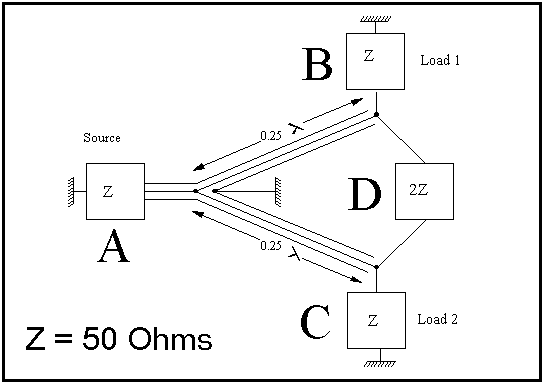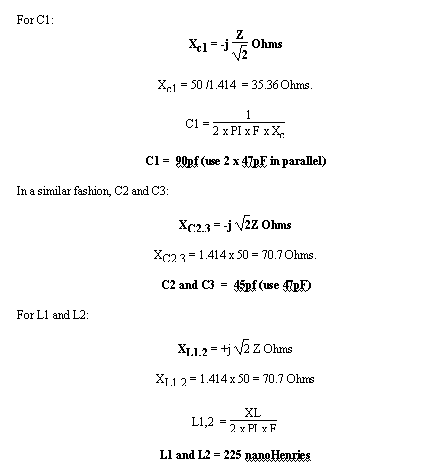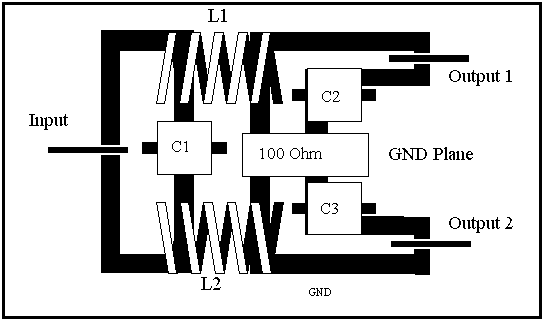# 50MHz Power SplittersIssue 33, April 1992 Six News

The use of power splitters and combiners is widespread in amateur radio but the design of these circuits is often considered to be a 'black art'. This short article does not attempt to be a treatise on the subject but concentrates on one particular type - the ubiquitous Wilkinson splitter/combiner. This is most commonly seen in amateur circles in use as the phasing harness in stacked antennas where there is a need to equally split the power from the transmitter between two antennas. The circuit diagram of the Wilkinson splitter/combiner is shown in Figure 1.Figure 1 - Circuit Diagram of the Wilkinson Power Divider

'Z' is the nominal input & output impedance, in this case 50 Ohms. If the circuit is used as a splitter, power is fed in via port 'A' and split through the use of lambda/4 coax between ports 'B' and 'C'. Each output is -3dB down on the input. When used as a combiner the output power is the sum of the two input powers (which should be identical), or +3dB. The 2Z (100 Ohm) resistor on the output, in effect port 'D', is used to absorb reflected power caused by imbalances in the divider. Of course, this resistor must be of a non-inductive variety.

The circuit is exactly the same whether it is used as a splitter or combiner. In the former, power flows from left to right, while in the later, it flows right to left.

The power splitting/combining is achieved through the use of two lengths of coaxial cable whose characteristic impedance is defined by:

Zo = square root (2) x Z

where Z is the input output impedances of the divider. i.e. for 50 Ohm coax:,

Zo = 1.414 x 50 = 70.7 Ohms.

The nearest standard impedance is 75 Ohm and cable of this impedance can be used with no problems. The length of the cable is 1/4 wavelength and can be calculated using the formula:

Length= 300 / (Frequency x 4 x velocity factor) metres

This results in a length of 990 mm at 50MHz, assuming a velocity of 0.66. A practical circuit diagram may be seen in Figure 2.Figure 2 - A Practical Coaxial Power Divider

Splitters and combiners can be used in many applications, two examples are outlined below. The first application shown in Figure 3 is one of the most common - a phasing harness for a 6M stacked beams. This example was first described by Richard Lax, G4AHN, in issue #23 of SIX NEWS.

Clearly for use as a phasing harness it is not possible to place a 100 Ohm resistor on the outputs as the 6M beams need to be stacked at least 12 feet apart. Also, for 50MHz, just quarter-wave elements would be too short! To solve this problem, one arm is made of length 0.75 lambda while the other is 1.75 lambda - but the principal of operation is exactly the same.Figure 3 - A 6M Phasing Harness

A second application is very useful for use in power amplifiers. Here two identical Wilkinson circuits are used to 'parallel' two similar amplifiers in a PA application (figure 4). An added benefit of this circuit is that if one amplifier goes down, half of the power of the other amplifier is fed to the load (-6dB). The other half is dissipated in the fourth port which, of course, is the 100 Ohm resistor. This is the only circumstance when high power will be dissipated in this resistor. The use of heavy UR57 (75 Ohm) and UR67 (50 Ohm) is obviously not of much use here! Better to use 2mm diameter RG179 (75 Ohm) and RG178 (50 Ohm).Figure 4 - Combining Two Amplifiers

The splitter / combiner can be built in a small Eddystone die-cast box with BNC sockets for the input and outputs(Figure 5). The two 990mm lambda/4 coax cables are rolled up inside the box with the 100 Ohm resistor connected across the two output sockets. Two boxes will be needed. It is not too important to fine tune the lambda/4 elements as there is no need for extreme accuracy. The bandwidth is 20% before a reflection coefficient of 5% is reached (SWR of 1.1:1).Figure 5 - Mounting the Coax Wilkinson Splitter/Combiner in a Diecast Box

The Wilkinson splitter using coaxial cable is ideal for use in high-power or antenna applications. But for use in low-power applications, like the PA described above, the use of coiled coax is cumbersome to say the least.Figure 6 - Lumped Wilkinson Splitter

If it is intended to build a PA that incorporates two paralleled transistors on a single PCB there must be a better way and indeed there is. This is a Lumped Component Wilkinson Splitter / Combiner shown in Figure 6. There is a price to pay for this size compaction and that is a narrower bandwidth. The bandwidth is only 10% for a VSWR of 1.1:1 but this hardly matters in narrow-band amateur applications.

For use on 50MHz the values of the various components are given by the following formulas:Figure 8 - The mathematics

Using the coil graphs in the back of the RSGB VHF/UHF Manual, this inductance equates to 5 turns of 18swg, 10mm inside diameter, spaced 1 wire diameter.

A recommended PCB layout is shown in Figure 7. Capacitors should be low-inductance SMT types and the resistor a non-inductive variety. If good quality capacitors are used then this circuit used as a combiner or a splitter can handle any power with ease. Examples have been built for HF that have worked up to 700 watts out.

The PCBs can either be placed in a separate boxes or even better used as part of a larger PCB containing the two paralleled amplifiers.Figure 7 - PCB Layout of Lumped Wilkinson Splitter / Combiner

The Wilkinson circuit is a most useful and indispensable circuit and I am sure that you will be able to think of uses other than the ones outlined in this article. Splitting an antenna between two receivers for example.

I wholeheartedly recommend that you read the article that this, together with many other varieties of hybrids are described in greater detail: 'Hybrid Networks and their uses in Radio-Frequency Circuits', R. G. Manton BSc (Eng), PhD, CEng, MIEE, The Radio and Electronic Engineer, Vol 54, No. 11/12, pp. 473-489, Nov., Dec. 1984.

73 Chris G3WOSTo return to the archive page click here